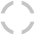Close Information
Description
•Spira Mirabilis

John T. Cooksey, ©2005, oil on canvas, 48in. diameter

Fibonacci series numbers 13 and 21 are the count of clockwise and counterclockwise spirals in this design.

The Spiral paintings are inspired by mathematical structures created by scientists studying the golden ratio, a seemingly magical geometric proportion commonly found in natural growth patterns. I have inserted a circle into each polygonal shape, using color to express simultaneous clockwise and counterclockwise mathematical spirals.

900,900
Direct Sale
•Spira Mirabilis 34-55

John T. Cooksey, ©2009, oil on canvas, 48in. diameter

Fibonacci series numbers 34 and 55 are the count of clockwise and counterclockwise spirals in this design.

The Spiral paintings are inspired by mathematical structures created by scientists studying the golden ratio, a seemingly magical geometric proportion commonly found in natural growth patterns. I have inserted a circle into each polygonal shape, using color to express simultaneous clockwise and counterclockwise mathematical spirals.

901,900
Direct Sale
•Spira Mirabilis 89-144

John T. Cooksey, ©2009, oil on canvas, 48in. diameter

Fibonacci series numbers 89 and 144 are the count of clockwise and counterclockwise spirals in this design.

The Spiral paintings are inspired by mathematical structures created by scientists studying the golden ratio, a seemingly magical geometric proportion commonly found in natural growth patterns. I have inserted a circle into each polygonal shape, using color to express simultaneous clockwise and counterclockwise mathematical spirals.

902,901
Direct Sale
•Bernoulli's Bubbles

John T. Cooksey, ©2005, oil on canvas, 48in. diameter

The Spiral paintings are inspired by mathematical structures created by scientists studying the golden ratio, a seemingly magical geometric proportion commonly found in natural growth patterns. I have inserted a circle into each polygonal shape, using color to express simultaneous clockwise and counterclockwise mathematical spirals.

900,900
Direct Sale
•Spira Omega

John T. Cooksey, ©2005, oil on canvas, 48in. diameter

Named for two opposing spirals that suggest the shape of a lower case omega.

The Spiral paintings are inspired by mathematical structures created by scientists studying the golden ratio, a seemingly magical geometric proportion commonly found in natural growth patterns. I have inserted a circle into each polygonal shape, using color to express simultaneous clockwise and counterclockwise mathematical spirals.

900,900
Direct Sale
Show Thumbnails
Show Thumbnails
Exit Stack View
Spiral Paintings

The Spiral paintings are inspired by mathematical structures created by scientists studying the golden ratio, a seemingly magical geometric proportion commonly found in natural growth patterns. I have inserted a circle into each polygonal shape, using color to express simultaneous clockwise and counterclockwise mathematical spirals.

Previous Image
Slide Show
Next Image
Spiral Paintings
Price Information
Full Screen Sound